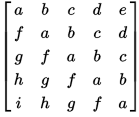# Check if given matrix is Toeplitz matrix or not

Given an NxN matrix, check if it is Toeplitz matrix or not. A Toeplitz matrix or diagonal-constant matrix is a matrix in which each descending diagonal from left to right is constant.

For instance, the following matrix is a Toeplitz matrix:Any N×N matrix A of the form is a Toeplitz matrix if
A(i, j) = A(i+1, j+1) = A(i+2, j+2) and so on..

Here, the i, j element of A is denoted A(i, j).

The idea is very simple. We traverse the matrix once and for each element `(i, j)`, we check if it is same as its immediate diagonal element `(i+1, j+1)` or not. If we found any element which differs from its immediate diagonal element, the matrix is not Toeplitz and we return false.

Output:

Toepliz matrix

## Java

Output:

Toepliz matrix

The time complexity of above solution is O(N2) and auxiliary space used by the program is O(1).(1 votes, average: 5.00 out of 5)Loading...